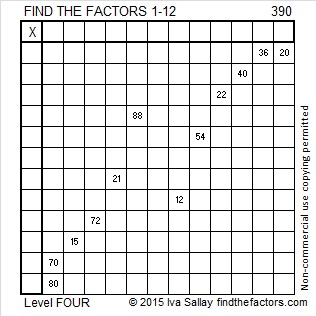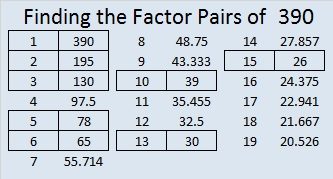# 390 and Level 4

390 is a multiple of 6 because it is even and all of its digits are divisible by 3. Scroll down past the puzzle to view its factors.Print the puzzles or type the factors on this excel file:12 Factors 2015-02-09

• 390 is a composite number.
• Prime factorization: 390 = 2 x 3 x 5 x 13
• The exponents in the prime factorization are 1, 1, 1, and 1. Adding one to each and multiplying we get (1 + 1)(1 + 1)(1 + 1)(1 + 1) = 2 x 2 x 2 x 2 = 16. Therefore 390 has exactly 16 factors.
• Factors of 390: 1, 2, 3, 5, 6, 10, 13, 15, 26, 30, 39, 65, 78, 130, 195, 390
• Factor pairs: 390 = 1 x 390, 2 x 195, 3 x 130, 5 x 78, 6 x 65, 10 x 39, 13 x 30, or 15 x 26
• 390 has no square factors that allow its square root to be simplified. √390 ≈ 19.748This site uses Akismet to reduce spam. Learn how your comment data is processed.